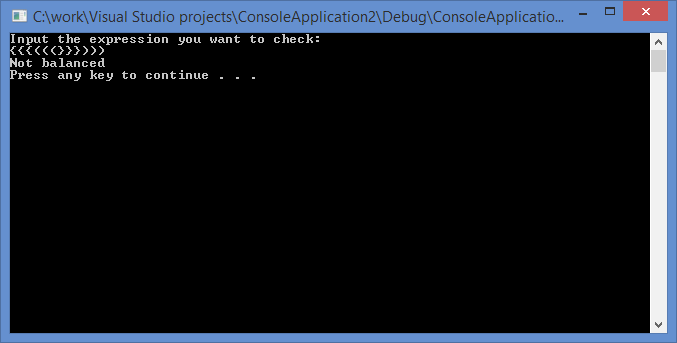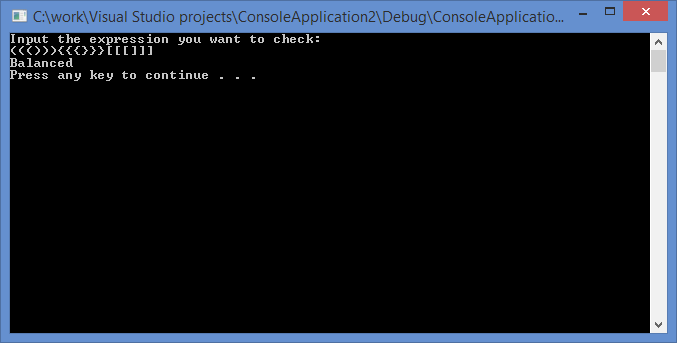# Web Assignment Sample: Check for Balanced Parentheses in an Expression

Hello! In this guide, I’ll show you the program to check the balance of different types of parentheses in an expression using C++.

Idea for Implementation

Everything would be much simpler if we had to check only one type of parentheses. If it is required to check the balance of the parentheses of only one type, for example, “(“ and “)”, we can read all the symbols one by one, ignoring all the symbols except the braces. If it is an opening bracket we increase the counter by 1, and if it’s a closing bracket we reduce the counter by one. After the check, it’s easy to define if the expression is balanced. If the counter is bigger than zero, we have more opening brackets. But if it goes lower than zero, we can’t check further. So we will use the stack.

Algorithm

1) Declare a stack.
2) Now iterate through the expression:
a) If the character is the starting bracket ‘(‘ or ‘{‘ or ‘[‘ then push it to the stack.
b) If the character is the closing bracket ‘)’ or ‘}’ or ‘]’ then pop it from the stack and match for relevant parenthesis. If it matches, pop it from the stack.
3) After iterations are complete, if there is a starting bracket left in the stack, then the parentheses are “not balanced”.

Screenshots of the working program:```#include<iostream>
#include<stdlib.h>
#include<stack>			//standard C++ data structure
using namespace std;

/*prororypes*/
bool Check(char e[]);
bool Coincide(char c1, char c2);

int main()
{
char a;

cout << "Input the expression you want to check: \n"; cin >> a;

if (Check(a))
printf("Balanced \n");
else
printf("Not balanced \n");

system("pause");

return 0;
}

bool Check(char e[])
{
/*stack variable*/
stack<char> Stack;
int i = 0;

/* iterate through the expression*/
while (e[i])
{

/*If the current element is an opening bracket then pust it to the stack*/
if (e[i] == '(' || e[i] == '{' || e[i] == '[')
{
Stack.push(e[i]);
}

/* If the current element is a closing bracket then check if the stack is
empty or it is its top element and pop it from the stack*/
if (e[i] == ')' || e[i] == '}' || e[i] == ']')
{
if (Stack.empty() || !Coincide(Stack.top(), e[i]))
{
return false;
}
else
{
Stack.pop();
}
}
i++;
}

/*If the stack is empty then the expression is balanced*/
return Stack.empty();
}

/* Coincide for relevent paranthesis */
bool Coincide(char c1, char c2)
{
if (c1 == '(' && c2 == ')')
return true;
else if (c1 == '{' && c2 == '}')
return true;
else if (c1 == '[' && c2 == ']')
return true;
else
return false;
}
```

Do you find our web assignment sample high quality? It was completed by an expert in the defined filed. If you are eager to complete the task of the same quality but you are having troubles with this apply for help to AssignmentShark. Our experts will offer assignment help you with any of your technical assignments. The only thing you need to do is to specify the requirements accurately. The rest of work you can rely on out experts. When using our service you have the ability to communicate with experts via live chat. If you need to complete your web assignment as soon as possible do not waste any minute! Make the order right away!

You can also be interested in GLSL shader examples posted on our blog.